SSC  >  Logical Reasoning - Test 5

# Logical Reasoning - Test 5

Test Description

## 25 Questions MCQ Test SSC CGL Tier 1 Mock Test Series | Logical Reasoning - Test 5

Logical Reasoning - Test 5 for SSC 2022 is part of SSC CGL Tier 1 Mock Test Series preparation. The Logical Reasoning - Test 5 questions and answers have been prepared according to the SSC exam syllabus.The Logical Reasoning - Test 5 MCQs are made for SSC 2022 Exam. Find important definitions, questions, notes, meanings, examples, exercises, MCQs and online tests for Logical Reasoning - Test 5 below.
Solutions of Logical Reasoning - Test 5 questions in English are available as part of our SSC CGL Tier 1 Mock Test Series for SSC & Logical Reasoning - Test 5 solutions in Hindi for SSC CGL Tier 1 Mock Test Series course. Download more important topics, notes, lectures and mock test series for SSC Exam by signing up for free. Attempt Logical Reasoning - Test 5 | 25 questions in 15 minutes | Mock test for SSC preparation | Free important questions MCQ to study SSC CGL Tier 1 Mock Test Series for SSC Exam | Download free PDF with solutions
 1 Crore+ students have signed up on EduRev. Have you?
Logical Reasoning - Test 5 - Question 1

### Select the related word from the given alternatives: Work : Experience :: Reading : ?

Detailed Solution for Logical Reasoning - Test 5 - Question 1

Experience is acquired from work. Similarly knowledge is acquired from reading.

Logical Reasoning - Test 5 - Question 2

### Select the related letters from the given alternatives: CNRX : EPTZ :: WHLD : ?

Detailed Solution for Logical Reasoning - Test 5 - Question 2

In CNRX : EPTZ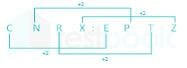Similarly,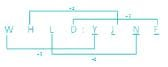“YJNF” related to “WHLD”

Hence, YJNF is correct option.

Logical Reasoning - Test 5 - Question 3

### Select the related numbers from the given alternatives: 33 : 27 :: 34 : ?

Detailed Solution for Logical Reasoning - Test 5 - Question 3

In 33 : 27
⇒ 33 = 27
Similarly, 34 = 81
Hence, 81 is correct option.

Logical Reasoning - Test 5 - Question 4

For the following question find the odd letter from the given alternatives:

Detailed Solution for Logical Reasoning - Test 5 - Question 4

Position of A = 1, I = 9, Y = 25, H = 8 are in English alphabet.
Now, A, I, and Y are at perfect square position in English alphabet but H is at perfect cube position in English alphabet.
Therefore, “H” is odd one among given alternatives.

Logical Reasoning - Test 5 - Question 5

In the following question, select the odd word from the given alternatives.

Detailed Solution for Logical Reasoning - Test 5 - Question 5

Number of Days in January, August and October are 31 but number of days in February is 28 or 29.

Logical Reasoning - Test 5 - Question 6

For the following question find the odd number from the given alternatives:

Detailed Solution for Logical Reasoning - Test 5 - Question 6

1) 16 = 4 × 4
21 = 3 x 7
HCF = 1

2) 12 = 2 × 3 × 2
27 = 3 × 3 × 3
HCF = 3

3) 15 = 3 × 5
28 = 7 × 4
HCF = 1

4)  14 = 2 × 7
45 = 3 × 3 × 5
HCF = 1
Therefore the odd numbers are 12 – 27 since for all other alternatives HCF is found to be 1.

Logical Reasoning - Test 5 - Question 7

Arrange the following words as per order in the dictionary:

1. Acknowledge 2. Application 3. Account 4. Arsenic 5. Arcad

Detailed Solution for Logical Reasoning - Test 5 - Question 7

According to the alphabetic order, the account comes first followed by acknowledge, application, arcade and arsenic.
Therefore, “3, 1, 2, 5, 4” is correct order as they will appear in dictionary order.

Logical Reasoning - Test 5 - Question 8

A series is given with one term missing. Choose the correct alternative from the given ones that will complete the series:
PWT, SVV, VUX, YTZ, BSB, ?

Detailed Solution for Logical Reasoning - Test 5 - Question 8

The pattern followed in seris is shown below,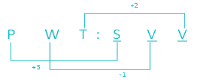Accordingly  S + 3 → V    V – 1 → U        V + 2 → X
V + 3 → Y   U – 1 → T        X + 2 → Z
Y + 3 → B   T – 1 → S         Z + 2 → B
B + 3 → E   S – 1 → R        B + 2 → D

Therefore, “ERD” is term which will complete the series.

Logical Reasoning - Test 5 - Question 9

A series is given with one term missing. Choose the correct alternative from the given ones that will complete the series:

24, 12, 36, ? , 54, 27

Detailed Solution for Logical Reasoning - Test 5 - Question 9

Pattern ⇒ ÷2, ×3, ÷2, ×3…
24 ÷ 2 = 12
12 × 3 = 36
36 ÷ 2 = 18
18 × 3 = 54
54 ÷ 2 = 27
The missing digit is 18.

Logical Reasoning - Test 5 - Question 10

Arun is the father of Cattie and Danny is the son of Bettie. Ernest is the brother of Arun. If Cattie is the sister of Danny. How is Bettie related to Ernest?

Detailed Solution for Logical Reasoning - Test 5 - Question 10

Below table shows symbol and their description,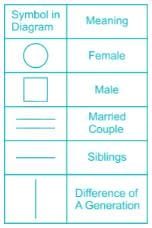1) Arun is the father of Cattie and Danny is the son of Bettie.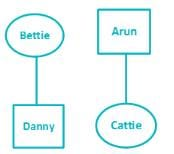2) Ernest is the brother of Arun.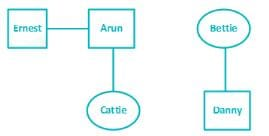3) Cattie is the sister of Danny. So Arun is the spouse of Bettie.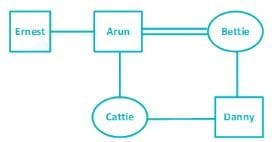Thus Bettie is Ernest’s sister in law

Logical Reasoning - Test 5 - Question 11

Arun, Bob, Cattie, Dannie, Ernest and Fred are sitting on the round table with equal distances. Fred is sitting opposite to Bob and between Arun and Ernest. Cattie is sitting opposite to Arun. Who are the neighbours of Ernest?

Detailed Solution for Logical Reasoning - Test 5 - Question 11

Possible solutions are: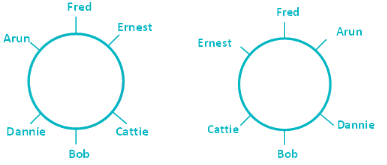The neighbours of Ernest are Fred and Cattie.

Logical Reasoning - Test 5 - Question 12

From the given alternative words, select the word which cannot be formed using the letters of the given word:

ENCYCLOPEDIA

Detailed Solution for Logical Reasoning - Test 5 - Question 12

CODPIECES cannot be formed using the letters since S is not present in the given word ENCYCLOPEDIA.

Logical Reasoning - Test 5 - Question 13

If 'TKU' stands for 'END' how will you code 'WEEKEND'?

Detailed Solution for Logical Reasoning - Test 5 - Question 13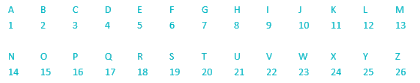T + E → 20 + 5 = 25
K + N → 11 +14 = 25
U + D → 21 + 4 = 25
W = 23 → 25 – 23 = 2 → B
E = 5 → 25 – 5 = 20 → T
E = 5 → 25 – 5 = 20 → T
K = 11 → 25 – 11 = 14 → N
E → T (Given)
N → K (Given)
D → U (Given)
WEEKEND → BTTNTKU
Therefore, “BTTNTKU” will be code for “WEEKEND”.

Logical Reasoning - Test 5 - Question 14

If '+' means '÷', '÷' means '–', '–' means 'x', 'x' means '+', then 44 + 4 ÷ 2 – 4 x 6 =

Detailed Solution for Logical Reasoning - Test 5 - Question 14

Based on condition given,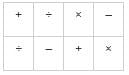⇒ 44 + 4 ÷ 2 – 4 x 6 = ?
⇒ 44 ÷ 4 – 2 x 4 + 6 = ?
⇒ 11 – 8 + 6 = ?
⇒ 9 = ?

Logical Reasoning - Test 5 - Question 15

In this question some equations are solved on the basis of a certain system. On the same basis find out the correct answer from amongst the four alternatives for the unsolved equation.

1 x 2 x 3 = 212
4 x 2 x 5 = 514
7 x 5 x 9 = 848
2 x 4 x 9 = ?

Detailed Solution for Logical Reasoning - Test 5 - Question 15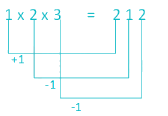Similarly 2 x 4 x 9 = 338.

Logical Reasoning - Test 5 - Question 16

Select the missing number in matrix from the given alternatives: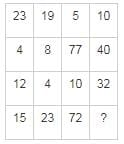Detailed Solution for Logical Reasoning - Test 5 - Question 16

23 + 4 – 12 = 15
19 + 8 – 4 = 23
5 + 77 – 10 = 72
10 + 40 – 32 = 18
Therefore, “18” is missing number in matrix.

Logical Reasoning - Test 5 - Question 17

Anita starts from a point walks 10 km towards South, turns left and walks 5 km, turns left again and walks. What is the direction she is facing now?

Detailed Solution for Logical Reasoning - Test 5 - Question 17

Direction diagram can be traced as shown below,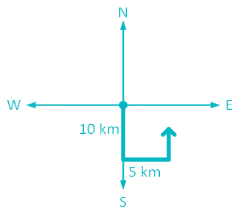Anita is facing North direction.

Logical Reasoning - Test 5 - Question 18

Consider the given statement/s to be true and decide which of the given conclusions/assumptions can definitely be drawn from the given statement.

Statement:
1. Some cats are dogs.
2. Some dogs are cows.
3. No cow is rat.

Conclusion
I. No rat is dog.
II. No cow is cat.
III. Some dogs are cats.

Detailed Solution for Logical Reasoning - Test 5 - Question 18

Venn diagram of given statement can be drawn as shown below,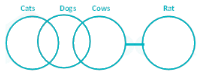Conclusion:
I. No rat is dog. ⇒ False (as there is possibility that some rat is dog)
II. No cow is cat. ⇒ False (as there is possibility that some cat is cow)
III. Some dogs are cats. True
From the above figures, it can be concluded that only III follows.

Logical Reasoning - Test 5 - Question 19

In the given cubes which colour is opposite to Red?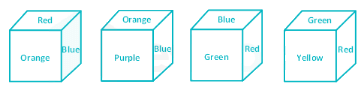Detailed Solution for Logical Reasoning - Test 5 - Question 19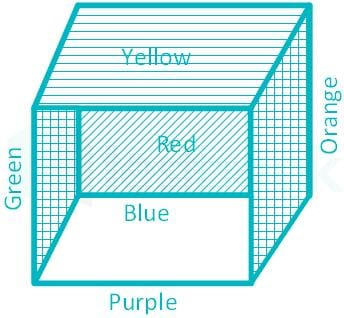Color opposite to Red is Purple.

Logical Reasoning - Test 5 - Question 20

Which of the following diagrams correctly represents Woman, doctors, professionals?

Detailed Solution for Logical Reasoning - Test 5 - Question 20

Some woman may be doctors also and both are professionals.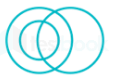Logical Reasoning - Test 5 - Question 21

Which answer figure will complete the pattern in the question figure?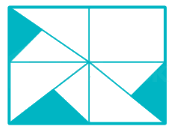Detailed Solution for Logical Reasoning - Test 5 - Question 21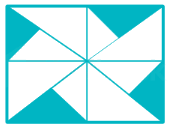Logical Reasoning - Test 5 - Question 22

From the given answer figures, select the one in which the question figure is hidden/embedded.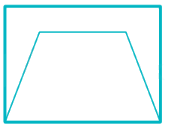Detailed Solution for Logical Reasoning - Test 5 - Question 22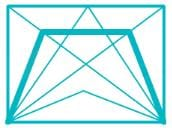Logical Reasoning - Test 5 - Question 23

A piece of transparent paper is folded. Find how the pattern appears when the paper is folded along the dotted line.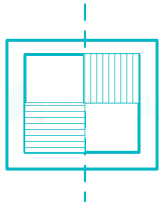Detailed Solution for Logical Reasoning - Test 5 - Question 23

The piece of paper will appear as shown when folded along the dotted line.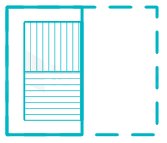Logical Reasoning - Test 5 - Question 24

If a mirror is placed on the line MN, then which of the answer figures is the right image of the given figure?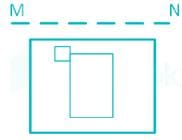Detailed Solution for Logical Reasoning - Test 5 - Question 24

The mirror image for the given figure is: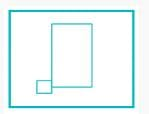Logical Reasoning - Test 5 - Question 25

In the question, a word is represented by only one set of numbers as given in any one of the alternatives. The sets of numbers given in the alternatives are represented by two classes of alphabets as in two matrices given below. The columns and rows of Matrix I are numbered from 0 to 4 and that of Matrix II are numbered from 5 to 9. A letter from these matrices can be represented first by its row and next by its column e.g. 'A' can be represented by 01, 13 etc. and 'S' can be represented by 55, 66 etc. Similarly, you have to identify the set for 'FEHTB'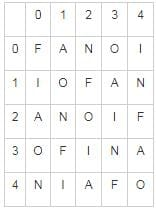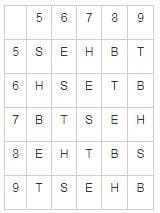Detailed Solution for Logical Reasoning - Test 5 - Question 25

1) 31, 21, 96, 87, 99 ⇒ F, N, S, T, B
2) 00, 56, 76, 68, 98 ⇒ F, E, T, T, H
3) 43, 85, 79, 87, 75 ⇒ F, E, H, T, B
4) 24, 56, 12, 59, 00 ⇒ F, E, F, T, F
Hence, 43, 85, 79, 87, 75 has the correct set for ‘FEHTB’.

## SSC CGL Tier 1 Mock Test Series

42 docs|103 tests
 Use Code STAYHOME200 and get INR 200 additional OFF Use Coupon Code
Information about Logical Reasoning - Test 5 Page
In this test you can find the Exam questions for Logical Reasoning - Test 5 solved & explained in the simplest way possible. Besides giving Questions and answers for Logical Reasoning - Test 5, EduRev gives you an ample number of Online tests for practice

## SSC CGL Tier 1 Mock Test Series

42 docs|103 tests